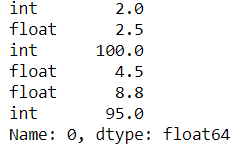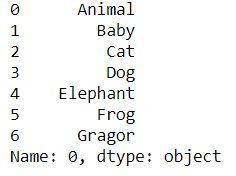# Pandas.DataFrame.iterrows() function in Python

Pandas DataFrame.iterrows() is used to iterate over a Pandas Dataframe rows in the form of (index, series) pair. This function iterates over the data frame column, it will return a tuple with the column name and content in form of a series.

## Pandas.DataFrame.iterrows() Syntax

Syntax: DataFrame.iterrows()

Yields:

• index- The index of the row. A tuple for a MultiIndex
• data- The data of the row as a Series

Returns: it: A generator that iterates over the rows of the frame

## Pandas DataFrame iterrows() Method

Sometimes we need to iter over the data frame rows and columns without using any loops, in this situation Pandas DataFrame.iterrows() plays a crucial role.

Example 1:

In the above example, we use Pandas DataFrame.iterrows() to iter over numeric data frame rows.

## Python3

 `import` `pandas as pd`   `# Creating a data frame along with column name` `df ``=` `pd.DataFrame([[``2``, ``2.5``, ``100``, ``4.5``, ``8.8``, ``95``]], columns``=``[` `                  ``'int'``, ``'float'``, ``'int'``, ``'float'``, ``'float'``, ``'int'``])`   `# Iter over the data frame rows` `# # using df.iterrows()` `itr ``=` `next``(df.iterrows())[``1``]` `itr`

Output:Example 2:

In the example, we iter over the data frame having no column names using Pandas DataFrame.iterrows()

## Python3

 `import` `pandas as pd`   `# Creating a data frame` `df ``=` `pd.DataFrame([[``'Animal'``, ``'Baby'``, ``'Cat'``, ``'Dog'``,` `                    ``'Elephant'``, ``'Frog'``, ``'Gragor'``]])`   `# Iterating over the data frame rows` `# using df.iterrows()` `itr ``=` `next``(df.iterrows())[``1``]` `itr`

Output :Note: As iterrows returns a Series for each row, it does not preserve dtypes across the rows.

Whether you're preparing for your first job interview or aiming to upskill in this ever-evolving tech landscape, GeeksforGeeks Courses are your key to success. We provide top-quality content at affordable prices, all geared towards accelerating your growth in a time-bound manner. Join the millions we've already empowered, and we're here to do the same for you. Don't miss out - check it out now!

Previous
Next